# 交易报告及短信通知的创建和发布

3 十二月 2013, 09:51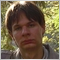0
1 324

### 1. 创建报告并通过 FTP 发送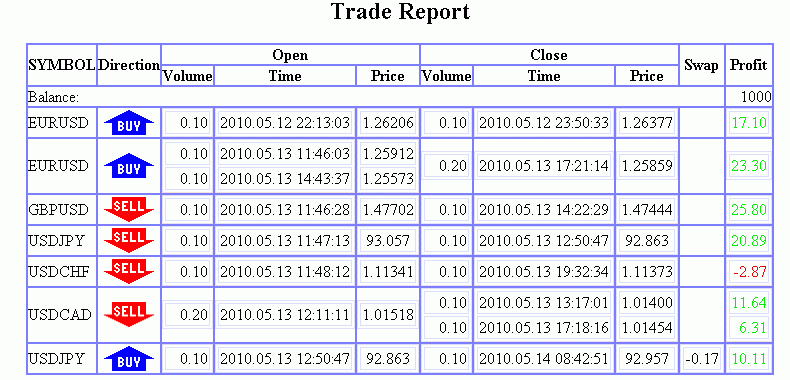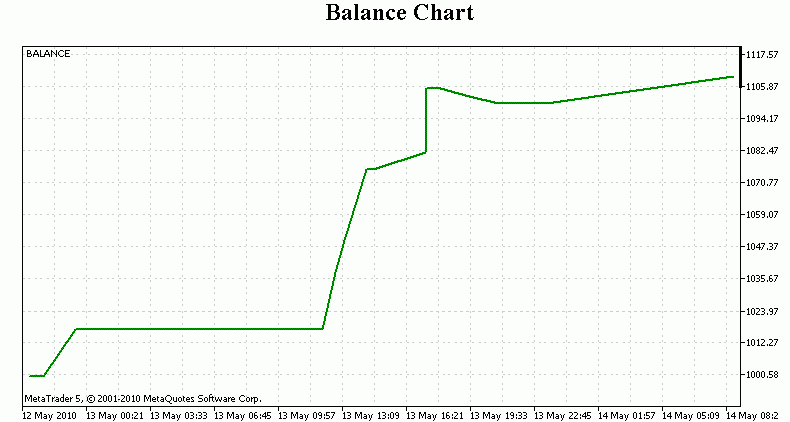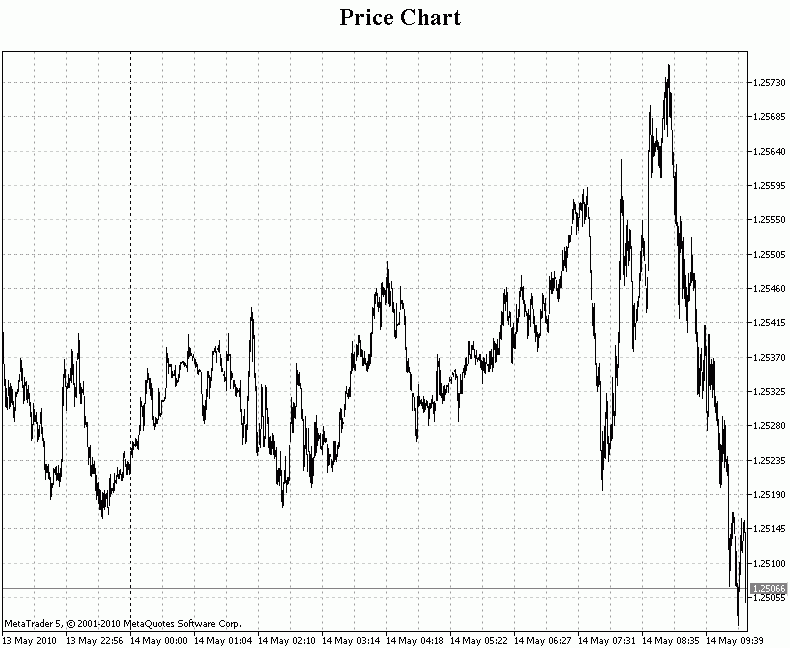symb_list[] 数组包含交易得以执行的金融工具名称的列表，而 lots_list[] 数组则包含交易处理时每个工具的开仓交易量。交易量正值对应的是买入持仓，而负值则对应着卖出持仓。如果交易量等于零，则意味着该工具没有敞口仓位。如果交易处理期间出现了未于列表中（symb_list[] 数组中）出现的金融工具 - 则将其添加到那里，且金融工具的编号（symb_total 变量）以 1 为增量增长。

```#define timeout 10           // 图表刷新时间
#define Picture1_width 800   // 报告中图表最大宽度
#define Picture2_width 800   // 报告中价格图表宽度
#define Picture2_height 600  // 报告中价格图表高度
#define Axis_Width 59        // 纵轴宽度 (以像素为单位)

```

```// 请求输入参数
#property script_show_inputs

```

```// 报告周期的枚举
enum report_periods
{
All_periods,
Last_day,
Last_week,
Last_month,
Last_year
};

```

```// 询问报告周期
input report_periods ReportPeriod=0;

```

```void OnStart()
{

```

```  datetime StartTime=0;           // 报告周期起始时间
datetime EndTime=TimeCurrent(); // 报告周期结束时间

// 计算报告周期起始时间
switch(ReportPeriod)
{
case 1:
StartTime=EndTime-86400;    // 日
break;
case 2:
StartTime=EndTime-604800;   // 周
break;
case 3:
StartTime=EndTime-2592000;  // 月
break;
case 4:
StartTime=EndTime-31536000; // 年
break;
}
// 如果不是以上的选项, 那么 StartTime=0 (整个周期)

```

```   int total_deals_number;  // 历史数据中的交易总数
int file_handle;         // 文件句柄
int i,j;                 // 循环计数器
int symb_total;          // 交易中的资产数量
int symb_pointer;        // 当前资产的指针
char deal_status[];      // 交易状态 (处理/未处理)
ulong ticket;            // 交易订单号
long hChart;             // 图表 id

double balance;           // 当前余额值
double balance_prev;      // 之前余额值
double lot_current;       // 当前交易交易量
double lots_list[];       // 根据资产种类的开放交易量列表
double current_swap;      // 当前交易的库存费
double current_profit;    // 当前交易的利润
double max_val,min_val;   // 最大值和最小值

string symb_list[];       // 交易的资产种类列表
string in_table_volume;   // 建仓交易量
string in_table_time;     // 进场时间
string in_table_price;    // 进场价格
string out_table_volume;  // 出场交易量
string out_table_time;    // 出场时间
string out_table_price;   // 出场价格
string out_table_swap;    // 出场库存费
string out_table_profit;  // 出场利润

bool symb_flag;           // 资产在列表中的标志

datetime time_prev;           // 前面的时间值
datetime time_curr;           // 当前时间值
datetime position_StartTime;  // 建仓时间
datetime position_EndTime;    // 最后一个退场时间

ENUM_TIMEFRAMES Picture1_period;  // 余额图表时段

```

``` // 开启一个新图表并设置其属性
hChart=ChartOpen(Symbol(),0);
ChartSetInteger(hChart,CHART_MODE,CHART_BARS);            // 柱状图
ChartSetInteger(hChart,CHART_AUTOSCROLL,true);            // 启用自动滚动
ChartSetInteger(hChart,CHART_COLOR_BACKGROUND,White);     // 背景色为白色
ChartSetInteger(hChart,CHART_COLOR_FOREGROUND,Black);     // 线和标签为黑色
ChartSetInteger(hChart,CHART_SHOW_OHLC,false);            // 不显示OHLC
ChartSetInteger(hChart,CHART_SHOW_BID_LINE,true);         // 显示 BID 线
ChartSetInteger(hChart,CHART_SHOW_LAST_LINE,false);       // 隐藏 LAST 线
ChartSetInteger(hChart,CHART_SHOW_GRID,true);             // 显示网格线
ChartSetInteger(hChart,CHART_SHOW_PERIOD_SEP,true);       // 显示时段分隔符
ChartSetInteger(hChart,CHART_COLOR_GRID,LightGray);       // 网格线为浅灰色
ChartSetInteger(hChart,CHART_COLOR_CHART_LINE,Black);     // 图表线为黑色
ChartSetInteger(hChart,CHART_COLOR_CHART_UP,Black);       // 向上的柱为黑色
ChartSetInteger(hChart,CHART_COLOR_CHART_DOWN,Black);     // 向下的柱为黑色
ChartSetInteger(hChart,CHART_COLOR_BID,Gray);             // BID 线为灰色
ChartSetInteger(hChart,CHART_COLOR_VOLUME,Green);         // 交易量和订单水平为绿色
ChartSetInteger(hChart,CHART_COLOR_STOP_LEVEL,Red);       // SL 和 TP 水平为红色
ChartSetString(hChart,CHART_COMMENT,ChartSymbol(hChart)); // 注释包含资产种类

```

```// 把图表保存为图像文件
ChartScreenShot(hChart,"picture2.gif",Picture2_width,Picture2_height);

```

```// 请求整个时段的交易历史
HistorySelect(0,TimeCurrent());

```

```// 打开图表文件
file_handle=FileOpen("report.html",FILE_WRITE|FILE_ANSI);

```

• html 文档的开头 (<html>)
• 带有背景色的 html 文档主体部分的开头 (<body bgcolor='#EFEFEF'>)
• 居中对齐 (<center>)
• 交易与仓位表的标题 (<h2>交易报告</h2>)
• 交易与仓位表的开头，带有对齐、边框宽度、背景颜色、边框颜色、单元格间距及单元格填充 (<table align='center' border='1' bgcolor='#FFFFFF' bordercolor='#7F7FFF' cellspacing='0' cellpadding='0'>)
• 表标题
```// 写 HTML 开头部分
FileWrite(file_handle,"<html>"+
"<body bgcolor='#EFEFEF'>"+
"<center>"+
"<table align='center' border='1' bgcolor='#FFFFFF' bordercolor='#7F7FFF' cellspacing='0' cellpadding='0'>"+
"<tr>"+
"<th rowspan=2>SYMBOL</th>"+
"<th rowspan=2>Direction</th>"+
"<th colspan=3>Open</th>"+
"<th colspan=3>Close</th>"+
"<th rowspan=2>Swap</th>"+
"<th rowspan=2>Profit</th>"+
"</tr>"+
"<tr>"+
"<th>Volume</th>"+
"<th>Time</th>"+
"<th>Price</th>"+
"<th>Volume</th>"+
"<th>Time</th>"+
"<th>Price</th>"+
"</tr>");

```

```// 历史中的交易数
total_deals_number=HistoryDealsTotal();

```

```// 设置资产列表，交易量列表和交易状态数组的维度
ArrayResize(symb_list,total_deals_number);
ArrayResize(lots_list,total_deals_number);
ArrayResize(deal_status,total_deals_number);

```

```// 把数组的所有原色设为0 - 交易没有被处理
ArrayInitialize(deal_status,0);

```

```balance=0;       // 初始余额
balance_prev=0;  // 之前余额

```

```// 列表中的资产数目
symb_total=0;

```

```// 处理历史中的所有交易
for(i=0;i<total_deals_number;i++)
{

```

```//选择交易，取得订单号
ticket=HistoryDealGetTicket(i);

```

```// 修改余额
balance+=HistoryDealGetDouble(ticket,DEAL_PROFIT);

```

```// 读取交易时间
time_curr=HistoryDealGetInteger(ticket,DEAL_TIME);

```

```// 如果是第一笔交易
if(i==0)
{
// 如果报告时段开始早于第一笔订单
// 则报告时段将从第一笔交易开始
if(StartTime<time_curr) StartTime=time_curr;
// 如果报告时段结束比当前时间晚,
// 则报告时段的结束时间等于当前时间
if(EndTime>TimeCurrent()) EndTime=TimeCurrent();
// 初始化最大最小余额
// 等于当前余额
max_val=balance;
min_val=balance;
// 根据报告时段计算余额图表
// 时间段
Picture1_period=PERIOD_M1;
if(EndTime-StartTime>(Picture1_width-Axis_Width)) Picture1_period=PERIOD_M2;
if(EndTime-StartTime>(Picture1_width-Axis_Width)*120) Picture1_period=PERIOD_M3;
if(EndTime-StartTime>(Picture1_width-Axis_Width)*180) Picture1_period=PERIOD_M4;
if(EndTime-StartTime>(Picture1_width-Axis_Width)*240) Picture1_period=PERIOD_M5;
if(EndTime-StartTime>(Picture1_width-Axis_Width)*300) Picture1_period=PERIOD_M6;
if(EndTime-StartTime>(Picture1_width-Axis_Width)*360) Picture1_period=PERIOD_M10;
if(EndTime-StartTime>(Picture1_width-Axis_Width)*600) Picture1_period=PERIOD_M12;
if(EndTime-StartTime>(Picture1_width-Axis_Width)*720) Picture1_period=PERIOD_M15;
if(EndTime-StartTime>(Picture1_width-Axis_Width)*900) Picture1_period=PERIOD_M20;
if(EndTime-StartTime>(Picture1_width-Axis_Width)*1200) Picture1_period=PERIOD_M30;
if(EndTime-StartTime>(Picture1_width-Axis_Width)*1800) Picture1_period=PERIOD_H1;
if(EndTime-StartTime>(Picture1_width-Axis_Width)*3600) Picture1_period=PERIOD_H2;
if(EndTime-StartTime>(Picture1_width-Axis_Width)*7200) Picture1_period=PERIOD_H3;
if(EndTime-StartTime>(Picture1_width-Axis_Width)*10800) Picture1_period=PERIOD_H4;
if(EndTime-StartTime>(Picture1_width-Axis_Width)*14400) Picture1_period=PERIOD_H6;
if(EndTime-StartTime>(Picture1_width-Axis_Width)*21600) Picture1_period=PERIOD_H8;
if(EndTime-StartTime>(Picture1_width-Axis_Width)*28800) Picture1_period=PERIOD_H12;
if(EndTime-StartTime>(Picture1_width-Axis_Width)*43200) Picture1_period=PERIOD_D1;
if(EndTime-StartTime>(Picture1_width-Axis_Width)*86400) Picture1_period=PERIOD_W1;
if(EndTime-StartTime>(Picture1_width-Axis_Width)*604800) Picture1_period=PERIOD_MN1;
// 改变开启的图表的时段
ChartSetSymbolPeriod(hChart,Symbol(),Picture1_period);
}

```

```else
// 如果不是第一笔交易
{
// 如果交易在图表周期之内, 绘制余额线,
// 并设置余额线的属性
if(time_curr>=StartTime && time_prev<=EndTime)
{
ObjectCreate(hChart,IntegerToString(i),OBJ_TREND,0,time_prev,balance_prev,time_curr,balance);
ObjectSetInteger(hChart,IntegerToString(i),OBJPROP_COLOR,Green);
// 如果线的两端都在报告周期之内,
// 将是粗线
if(time_prev>=StartTime && time_curr<=EndTime)
ObjectSetInteger(hChart,IntegerToString(i),OBJPROP_WIDTH,2);
}
// 如果余额的新值超过
// 最大值或最小值范围, 必须做调整
if(balance<min_val) min_val=balance;
if(balance>max_val) max_val=balance;
}

```

```// 修改前一时间值
time_prev=time_curr;

```

```// 如果交易还没有被处理
if(deal_status[i]<127)
{

```

```// 如果交易为余额变动
if(HistoryDealGetInteger(ticket,DEAL_TYPE)==DEAL_TYPE_BALANCE)
{
// 如果在报告周期内 - 在报告中写下对应描述
if(time_curr>=StartTime && time_curr<=EndTime)
FileWrite(file_handle,"<tr><td colspan='9'>Balance:</td><td align='right'>",HistoryDealGetDouble(ticket,DEAL_PROFIT),
"</td></tr>");
// 把交易标记为已处理
deal_status[i]=127;
}

```

```// 如果交易为买入或卖出
{
// 检查列表中有无此交易的资产类型
symb_flag=false;
for(j=0;j<symb_total;j++)
{
if(symb_list[j]==HistoryDealGetString(ticket,DEAL_SYMBOL))
{
symb_flag=true;
symb_pointer=j;
}
}
// 如果列表中没有此资产
if(symb_flag==false)
{
symb_list[symb_total]=HistoryDealGetString(ticket,DEAL_SYMBOL);
lots_list[symb_total]=0;
symb_pointer=symb_total;
symb_total++;
}

```

```// 设置交易开始时间的初始值
position_StartTime=time_curr;
// 设置交易结束时间的初始值
position_EndTime=time_curr;

```

in_table_volume、in_table_time、in_table_price、out_table_volume、out_table_time、out_table_price、out_table_swap 和 out_table_profit 变量则会存储将加入更大表格单元格内的表格：进入市场的交易量、时间和价格；退出市场的交易量、时间、价格、掉期和利润。in_table_volume 变量还会存储金融工具的名称和到某图像的链接（对应敞口仓位方向）。为上述所有变量赋初始值。

```

// 创建报告中的字符串 - 金融工具, 仓位方向, 进入市场的交易量表格起始
symb_list[symb_pointer],"</td><td align='center'><img src='buy.gif'></td><td><table border='1' width='100%' bgcolor='#FFFFFF'
bordercolor='#DFDFFF'>");
if(HistoryDealGetInteger(ticket,DEAL_TYPE)==DEAL_TYPE_SELL) StringConcatenate(in_table_volume,"<tr><td align='left'>",
symb_list[symb_pointer],"</td><td align='center'><img src='sell.gif'></td><td><table border='1' width='100%' bgcolor='#FFFFFF'
bordercolor='#DFDFFF'>");
// 创建进入市场的时间表格起始
in_table_time="<td><table border='1' width='100%' bgcolor='#FFFFFF' bordercolor='#DFDFFF'>";
// 创建进入市场的价格表格起始
in_table_price="<td><table border='1' width='100%' bgcolor='#FFFFFF' bordercolor='#DFDFFF'>";
// 创建退出市场的交易量表格起始
out_table_volume="<td><table border='1' width='100%' bgcolor=#FFFFFF bordercolor='#DFDFFF'>";
// 创建退出市场的时间表格起始
out_table_time="<td><table border='1' width='100%' bgcolor='#FFFFFF' bordercolor='#DFDFFF'>";
// 创建退出市场的价格表格起始
out_table_price="<td><table border='1' width='100%' bgcolor='#FFFFFF' bordercolor='#DFDFFF'>";
// 创建退出市场的库存费表格起始
out_table_swap="<td><table border='1' width='100%' bgcolor=#FFFFFF bordercolor='#DFDFFF'>";
// 创建退出市场的利润表格起始
out_table_profit="<td><table border='1' width='100%' bgcolor=#FFFFFF bordercolor='#DFDFFF'>";

```

```// 处理此仓位从现在开始的所有交易(直到平仓)
for(j=i;j<total_deals_number;j++)
{
// 如果交易还没有被处理 - 处理它
if(deal_status[j]<127)
{

```

```// 选择交易, 取得订单号
ticket=HistoryDealGetTicket(j);

```

```// 如果交易工具和仓位工具相匹配, 则进行处理
if(symb_list[symb_pointer]==HistoryDealGetString(ticket,DEAL_SYMBOL))
{
// 取得交易时间
time_curr=HistoryDealGetInteger(ticket,DEAL_TIME);
// 如果交易时间超出了仓位时间范围
// - 扩展仓位时间
if(time_curr<position_StartTime) position_StartTime=time_curr;
if(time_curr>position_EndTime) position_EndTime=time_curr;
// 取得交易的交易量
lot_current=HistoryDealGetDouble(ticket,DEAL_VOLUME);

```

```// 如果交易是买入
{

```

```// 如果仓位是卖出 - 这将会从市场退出
if(NormalizeDouble(lots_list[symb_pointer],2)<0)
{
// 如果买入交易量大于开启的卖出持仓交易量, 这就是in/out
if(NormalizeDouble(lot_current+lots_list[symb_pointer],2)>0)
{
// 创建退出市场的交易量表格 - 只指出开启的卖出持仓交易量
StringConcatenate(out_table_volume,out_table_volume,"<tr><td align='right'>",DoubleToString(-lots_list[symb_pointer],2),"</td></tr>");
// 把仓位标记为部分处理
deal_status[j]=1;
}
else
{
// 如果买入交易量小于等于卖出持仓 - 则是部分或全部平仓
// 创建退出市场的交易量表格
StringConcatenate(out_table_volume,out_table_volume,"<tr><td align='right'>",DoubleToString(lot_current,2),"</td></tr>");
// 把交易标记为已处理
deal_status[j]=127;
}

// 创建退出市场的时间表格
StringConcatenate(out_table_time,out_table_time,"<tr><td align='center'>",TimeToString(time_curr,TIME_DATE|TIME_SECONDS),"</td></tr>");

// 创建退出市场的价格表格
StringConcatenate(out_table_price,out_table_price,"<tr><td align='center'>",DoubleToString(HistoryDealGetDouble(ticket,DEAL_PRICE),
(int)SymbolInfoInteger(symb_list[symb_pointer],SYMBOL_DIGITS)),"</td></tr>");

// 取得当前交易的库存费
current_swap=HistoryDealGetDouble(ticket,DEAL_SWAP);

// 如果库存费等于0 - 创建退出市场库存费表格的空字符串
if(NormalizeDouble(current_swap,2)==0) StringConcatenate(out_table_swap,out_table_swap,"<tr></tr>");
// 否则创建退出市场的库存费表格的库存费字符串
else StringConcatenate(out_table_swap,out_table_swap,"<tr><td align='right'>",DoubleToString(current_swap,2),"</td></tr>");

// 取得当前交易的利润
current_profit=HistoryDealGetDouble(ticket,DEAL_PROFIT);

// 如果利润为负 (亏损) - 在退出市场的利润表格中显示为红色
if(NormalizeDouble(current_profit,2)<0) StringConcatenate(out_table_profit,out_table_profit,"<tr><td align=right><SPAN style='COLOR: #EF0000'>",
DoubleToString(current_profit,2),"</SPAN></td></tr>");
// 否则 - 显示为绿色
else StringConcatenate(out_table_profit,out_table_profit,"<tr><td align='right'><SPAN style='COLOR: #00EF00'>",
DoubleToString(current_profit,2),"</SPAN></td></tr>");
}

```

```else
// 如果持仓为买入 - 这将是进入市场
{
// 如果交易已经被部分处理 (in/out)
if(deal_status[j]==1)
{
// 创建进入市场的交易量表格 (交易量, 是否为 in/out 之后, 写在此处)
StringConcatenate(in_table_volume,in_table_volume,"<tr><td align='right'>",DoubleToString(lots_list[symb_pointer],2),"</td></tr>");
// 将会产生交易量改变的补偿,  (交易的交易量已经考虑在内)
lots_list[symb_pointer]-=lot_current;
}
// 如果交易还没有被处理
else StringConcatenate(in_table_volume,in_table_volume,"<tr><td align='right'>",DoubleToString(lot_current,2),"</td></tr>");

// 创建进入市场的时间表格
StringConcatenate(in_table_time,in_table_time,"<tr><td align center>",TimeToString(time_curr,TIME_DATE|TIME_SECONDS),"</td></tr>");

// 进入市场的价格表格
StringConcatenate(in_table_price,in_table_price,"<tr><td align='center'>",DoubleToString(HistoryDealGetDouble(ticket,DEAL_PRICE),
(int)SymbolInfoInteger(symb_list[symb_pointer],SYMBOL_DIGITS)),"</td></tr>");

// 把交易标记为已处理
deal_status[j]=127;
}

```

``` // 根据当前资产类型修改仓位交易量, 考虑当前交易的交易量
lots_list[symb_pointer]+=lot_current;
// 如果当前资产类型的仓位交易量变成0 - 已经平仓
if(NormalizeDouble(lots_list[symb_pointer],2)==0 || deal_status[j]==1) break;
}

```

```       // 如果交易是卖出
if(HistoryDealGetInteger(ticket,DEAL_TYPE)==DEAL_TYPE_SELL)
{
// 如果仓位已经建立买入 - 这将会退出市场
if(NormalizeDouble(lots_list[symb_pointer],2)>0)
{
// 如果卖出交易量大于买入持仓交易量 - 这是 in/out
if(NormalizeDouble(lot_current-lots_list[symb_pointer],2)>0)
{
// 创建退出市场的交易量表格 - 只指明买入持仓交易量
StringConcatenate(out_table_volume,out_table_volume,"<tr><td align='right'>",DoubleToString(lots_list[symb_pointer],2),"</td></tr>");
// 把仓位标记为部分处理
deal_status[j]=1;
}
else
{
// 如果卖出交易量大于开启的卖出仓位交易量 - 这是部分或全部平仓
// 创建退出市场的交易量表格
StringConcatenate(out_table_volume,out_table_volume,"<tr><td align='right'>",DoubleToString(lot_current,2),"</td></tr>");
// 把交易标记为已处理
deal_status[j]=127;
}

// 创建退出市场的时间表格
StringConcatenate(out_table_time,out_table_time,"<tr><td align='center'>",TimeToString(time_curr,TIME_DATE|TIME_SECONDS),"</td></tr>");

// 创建退出市场的价格表格
StringConcatenate(out_table_price,out_table_price,"<tr><td align='center'>",DoubleToString(HistoryDealGetDouble(ticket,DEAL_PRICE),
(int)SymbolInfoInteger(symb_list[symb_pointer],SYMBOL_DIGITS)),"</td></tr>");

// 取得当前交易的库存费
current_swap=HistoryDealGetDouble(ticket,DEAL_SWAP);

// 如果库存费等于0 - 创建退出市场库存费表格的空字符串
if(NormalizeDouble(current_swap,2)==0) StringConcatenate(out_table_swap,out_table_swap,"<tr></tr>");
// 否则创建退出市场的库存费表格的库存费字符串
else StringConcatenate(out_table_swap,out_table_swap,"<tr><td align='right'>",DoubleToString(current_swap,2),"</td></tr>");

// 取得当前交易的利润
current_profit=HistoryDealGetDouble(ticket,DEAL_PROFIT);

// 如果利润为负 (亏损) - 在退出市场的利润表格中显示为红色
if(NormalizeDouble(current_profit,2)<0) StringConcatenate(out_table_profit,out_table_profit,"<tr><td align='right'>
<SPAN style='COLOR: #EF0000'>",DoubleToString(current_profit,2),"</SPAN></td></tr>");
// 否则 - 显示为绿色
else StringConcatenate(out_table_profit,out_table_profit,"<tr><td align='right'><SPAN style='COLOR: #00EF00'>",
DoubleToString(current_profit,2),"</SPAN></td></tr>");
}
else
// 如果是卖出仓位 - 这将是进入市场
{
// 如果交易已经被部分处理 (in/out)
if(deal_status[j]==1)
{
// 创建进入市场的交易量表格 (交易量, 是否为 in/out 之后, 写在此处)
StringConcatenate(in_table_volume,in_table_volume,"<tr><td align='right'>",DoubleToString(-lots_list[symb_pointer],2),"</td></tr>");

// 将会产生交易量改变的补偿,  (交易的交易量已经考虑在内)
lots_list[symb_pointer]+=lot_current;
}
// 如果交易还没有被处理
else StringConcatenate(in_table_volume,in_table_volume,"<tr><td align='right'>",DoubleToString(lot_current,2),"</td></tr>");

// 创建进入市场的时间表格
StringConcatenate(in_table_time,in_table_time,"<tr><td align='center'>",TimeToString(time_curr,TIME_DATE|TIME_SECONDS),"</td></tr>");

// 进入市场的价格表格
StringConcatenate(in_table_price,in_table_price,"<tr><td align='center'>",DoubleToString(HistoryDealGetDouble(ticket,DEAL_PRICE),
(int)SymbolInfoInteger(symb_list[symb_pointer],SYMBOL_DIGITS)),"</td></tr>");

// 把交易标记为已处理
deal_status[j]=127;
}
// 根据当前资产类型修改仓位交易量, 考虑当前交易的交易量
lots_list[symb_pointer]-=lot_current;
// 如果当前资产类型的仓位交易量变成0 - 已经平仓
if(NormalizeDouble(lots_list[symb_pointer],2)==0 || deal_status[j]==1) break;
}
}
}
}

```

``` // 如果仓位时间在报告时间之内- 仓位会打印到报告中
if(position_EndTime>=StartTime && position_StartTime<=EndTime) FileWrite(file_handle,
in_table_volume,"</table></td>",
in_table_time,"</table></td>",
in_table_price,"</table></td>",
out_table_volume,"</table></td>",
out_table_time,"</table></td>",
out_table_price,"</table></td>",
out_table_swap,"</table></td>",
out_table_profit,"</table></td></tr>");```

```   }
// 修改余额
balance_prev=balance;
}

```

```// 创建 html-文件的末尾
FileWrite(file_handle,"</table><br><br><h2>Balance Chart</h2><img src='picture1.gif'><br><br><br><h2>Price Chart</h2><img src='picture2.gif'></center></body></html>");
// 关闭文件
FileClose(file_handle);

```

```// 取得当前时间
time_curr=TimeCurrent();
// 等待图表更新
while(SeriesInfoInteger(Symbol(),Picture1_period,SERIES_BARS_COUNT)==0 && TimeCurrent()-time_curr<timeout) Sleep(1000);

```

```// 设置平衡图表的最大值和最小值 (上下边界有10% 缩进)
ChartSetDouble(hChart,CHART_FIXED_MAX,max_val+(max_val-min_val)/10);
ChartSetDouble(hChart,CHART_FIXED_MIN,min_val-(max_val-min_val)/10);

```

```// 设置平衡图表的属性
ChartSetInteger(hChart,CHART_MODE,CHART_LINE);                // 图表为线形图
ChartSetInteger(hChart,CHART_FOREGROUND,false);               // 图表在前
ChartSetInteger(hChart,CHART_SHOW_BID_LINE,false);            // 隐藏 BID 线
ChartSetInteger(hChart,CHART_COLOR_VOLUME,White);             // 交易量和订单水平为白色
ChartSetInteger(hChart,CHART_COLOR_STOP_LEVEL,White);         // 止损和获利水平为白色
ChartSetInteger(hChart,CHART_SHOW_GRID,true);                 // 显示网格
ChartSetInteger(hChart,CHART_COLOR_GRID,LightGray);           // 网格为浅灰色
ChartSetInteger(hChart,CHART_SHOW_PERIOD_SEP,false);          // 隐藏周期分隔
ChartSetInteger(hChart,CHART_SHOW_VOLUMES,CHART_VOLUME_HIDE); // 隐藏交易量
ChartSetInteger(hChart,CHART_COLOR_CHART_LINE,White);         // 图表为白色
ChartSetInteger(hChart,CHART_SCALE,0);                        // 最小尺度
ChartSetInteger(hChart,CHART_SCALEFIX,true);                  // 纵轴固定尺度
ChartSetInteger(hChart,CHART_SHIFT,false);                    // 没有图表转换
ChartSetInteger(hChart,CHART_AUTOSCROLL,true);                // 启用自动滚动
ChartSetString(hChart,CHART_COMMENT,"BALANCE");               // 图表的注释

```

```// 重绘平衡图表
ChartRedraw(hChart);
Sleep(8000);

```

```// 平衡图表截图
ChartScreenShot(hChart,"picture1.gif",(int)(EndTime-StartTime)/PeriodSeconds(Picture1_period),
(int)(EndTime-StartTime)/PeriodSeconds(Picture1_period)/2,ALIGN_RIGHT);

```

```// 删除平衡图表的所有对象
ObjectsDeleteAll(hChart);
// 关闭图表
ChartClose(hChart);

```

```// 如果启用了发布报告 - 通过 FTP 发送
// HTML-文件和两个图片 - 价格图表和平衡图表
if(TerminalInfoInteger(TERMINAL_FTP_ENABLED))
{
SendFTP("report.html");
SendFTP("picture1.gif");
SendFTP("picture2.gif");
}
}

```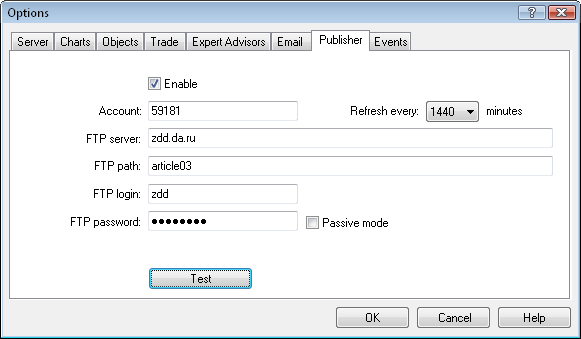### 2. 以短消息形式向手机发送通知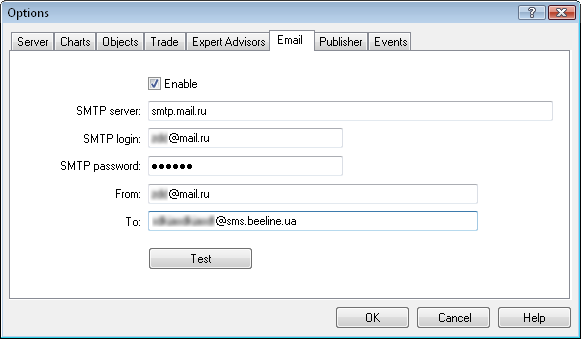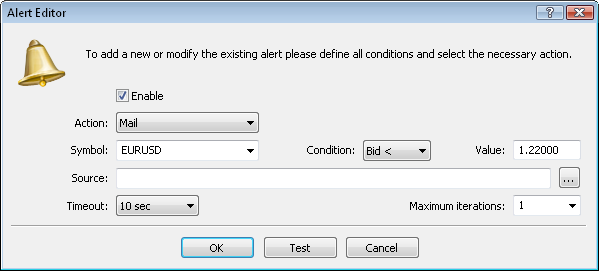```OrderSend(request,result};

```

换上

```string msg_subj,msg_text;
if(OrderSend(request,result))
{
switch(request.action)
{
switch(request.type)
{
StringConcatenate(msg_text,"Buy ",result.volume," ",request.symbol," 价位 ",result.price,", 止损=",request.sl,", 获利=",request.tp);
break;
case ORDER_TYPE_SELL:
StringConcatenate(msg_text,"Sell ",result.volume," ",request.symbol," 价位 ",result.price,", 止损=",request.sl,", 获利=",request.tp);
break;
}
break;
switch(request.type)
{
StringConcatenate(msg_text,"设置BuyLimit ",result.volume," ",request.symbol," 价位 ",request.price,", 止损=",request.sl,", 获利=",request.tp);
break;
case ORDER_TYPE_SELL_LIMIT:
StringConcatenate(msg_text,"设置 SellLimit ",result.volume," ",request.symbol," 价位 ",request.price,", 止损=",request.sl,", 获利=",request.tp);
break;
StringConcatenate(msg_text,"设置 BuyStop ",result.volume," ",request.symbol," 价位 ",request.price,", 止损=",request.sl,", 获利=",request.tp);
break;
case ORDER_TYPE_SELL_STOP:
StringConcatenate(msg_text,"设置 SellStop ",result.volume," ",request.symbol," 价位 ",request.price,", 止损=",request.sl,", 获利=",request.tp);
break;
StringConcatenate(msg_text,"设置 BuyStopLimit ",result.volume," ",request.symbol," 价位 ",request.price,", stoplimit=",request.stoplimit,
", 止损=",request.sl,", 获利=",request.tp);
break;
case ORDER_TYPE_SELL_STOP_LIMIT:
StringConcatenate(msg_text,"设置 SellStop ",result.volume," ",request.symbol," 价位 ",request.price,", stoplimit=",request.stoplimit,
", 止损=",request.sl,", 获利=",request.tp);
break;
}
break;
StringConcatenate(msg_text,"修改止损和获利. SL=",request.sl,", TP=",request.tp);
break;
StringConcatenate(msg_text,"修改订单",result.price,", 止损=",request.sl,", 获利=",request.tp);
break;
msg_text="删除订单";
break;
}
}
else msg_text="错误!";
SendMail(msg_subj,msg_text);

```

OrderSend() 函数会在交易请求后，利用 SendMail() 函数发送一条消息。其中包含交易账号、经纪人名称以及实施动作（买入、卖出、下达挂单、订单修改或删除）的相关信息，如下所示：

```59181-MetaQuotes Software Corp. Buy 0.1 EURUSD at price 1.23809, SL=1.2345, TP=1.2415

```

```void OnInit()
{
EventSetTimer(3600);
}

```

在 OnDeinit() 中，不要忘了用 EventKillTimer() 将其关闭：

```void OnDeinit(const int reason)
{
EventKillTimer();
}

```

```void OnTimer()
{
SendMail(Symbol(),DoubleToString(SymbolInfoDouble(Symbol(),SYMBOL_BID),_Digits));
}

```

### 总结

report.zip (33.08 KB)
sendreport_en.mq5 (52.91 KB)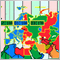一个基于不同大陆不同时区的交易策略实例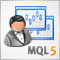MQL5：在 MetaTrader 5 中分析和处理商品期货交易委员会 (CFTC) 报告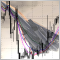MQL5 中的事件处理：快速更改 MA 周期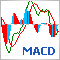创建具有图形控制选项的指标